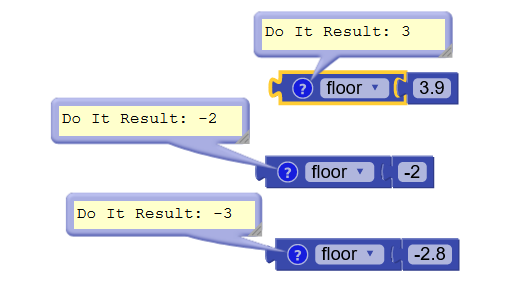# Maths function problem discussion

i want to use greatest integer function in my app, how can i do that

for those who don’t know what it is, it is a function which returns the greatest integer. for an example -

 = 3

[3.2] = 3

[3.9] = 3

[-2] = -2

[-2.8] = -3

Use floor from math blocks1 Like

3.9=3
But
-2.8=-3 how??see this graph

1 Like

The Greatest Integer Function is also known as the Floor Function . · It is written as f(x)=⌊x⌋. · The value of ⌊x⌋ is the largest integer that is less than or equal to x

2 Likes

Floor returns least integer value.
3 < 3.9 and -3 < -2.8

Ceiling would have returned 4 for 3.9 and -2 for -2.8

3 Likes

Learnt new concept. Sorry, i have never used that . Thank you @vknow360 ( 360)

1 Like

This topic was automatically closed 30 days after the last reply. New replies are no longer allowed.